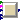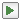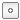## Buildings.Controls.Predictors

Package with models for load prediction

### Information

This package contains components models for load prediction.

Extends from Modelica.Icons.Package (Icon for standard packages).

### Package Content

Name DescriptionElectricalLoad Block that predicts an electrical loadTypes Types for prediction modelsExamples Collection of models that illustrate model use and test modelsValidation Collection of models that validate the load predictorsBaseClasses Package with base classes

##Buildings.Controls.Predictors.ElectricalLoad

Block that predicts an electrical load### Information

Data-driven model that predicts the electrical load. This load prediction can for example be used in a demand response client.

The model computes either an average baseline or a linear regression with respect to outside temperature. For both, optionally a day-of adjustment can be made.

#### Computation of baseline

Separate loads are computed for any types of days. The type of day is an input signal received from the connector `typeOfDay`, and must be equal to any value defined in Buildings.Controls.Types.Day. This input is a vector where the first element corresponds to the current day, the next element to tomorrow, and so on. The dimension of this input vector is typically 2 if the demand is to be predicted for the next 24 hours. If it is for the next 48 hours, then the dimension is 3. Using a vector is required as the prediction could be from noon of a workday to noon of a holiday or week-end day.

The average baseline is the average of the consumed power of the previous nhis days for the same time interval. The default value is nhis=10 days. For example, if the prediction is mode for 1 hour time windows, then there are 24 baseline values for each day, each being the average power consumed in the past 10 days that have the same `typeOfDay`.

The linear regression model computes the predicted power as a linear function of the current outside temperature. The two coefficients for the linear function are obtained using a regression of the past nhis days.

If the input signal `storeHistory` is `true`, then the prediction is no longer carried out for this day until midnight. For example, if used for a demand respond client, on an event day, one may want to set `storeHistory=true` when the building operates in demand respond mode. Then any time interval after this signal is received is excluded from the baseline computation. Storing history terms for the baseline resumes automatically at midnight.

If no history term is present for the current time interval and the current type of day, then the predicted power consumption `PPre[:]` will be zero.

If the parameter `use_dayOfAdj = true`, then the day-of adjustment is computed. (Some literature call this morning-of adjustment, but we call it day-of adjustment because the adjustment can also be in the afternoon if the peak is in the late afternoon hours.) The day-of adjustment can be used with any of the above baseline computations. The parameters `dayOfAdj_start` and `dayOfAdj_end` determine the time window during which the day-of adjustment is computed. Both need to be negative times, measured in seconds prior to the time at which the power consumption is predicted. For example, to use a day-of adjustment for the window of 4 to 1 hours prior to the event time, set `dayOfAdj_start=-4*3600` and `dayOfAdj_end=-3600`.

The day-of adjustment is computed as follows: First, the average power Pave consumed over the day-of time window is computed. Next, the average power Phis is computed for the past nhis days. Then, the adjustment factor is computed as

a = min(amax, max(amin, Pave ⁄ Phis),

where amin and amax are the minimum and maximum adjustment factors as defined by the parameters `adjFacMin` and `adjFacMax`.

Extends from Modelica.Blocks.Icons.DiscreteBlock (Graphical layout of discrete block component icon).

### Parameters

TypeNameDefaultDescription
IntegernSam24Number of intervals in a day for which baseline is computed
IntegernPre1Number of intervals for which future load need to be predicted (set to one to only predict current time, or to nSam to predict one day)
IntegernHis10Number of history terms to be stored
Booleanuse_dayOfAdjtrueif true, use the day of adjustment
TimedayOfAdj_start-14400Number of hours prior to current time when day of adjustment starts [s]
TimedayOfAdj_end-3600Number of hours prior to current time when day of adjustment ends [s]

### Connectors

TypeNameDescription
input RealInputTOutOutside air temperature [K]
input RealInputTOutFut[nPre - 1]Future outside air temperatures [K]
input RealInputEConConsumed electrical energy [J]
output RealOutputPPre[nPre]Predicted power consumptions (first element is for current time [W]
input DayTypeInputtypeOfDay[integer((nPre - 1)/nSam) + 2]Type of day for the current and the future days for which a prediction is to be made. Typically, this has dimension 2 for predictions up to and including 24 hours, and 2+n for any additional day
input BooleanInputstoreHistoryIf false, history terms are no longer stored for the remainder of the day